# What is OpenCV’s INTER_AREA Actually Doing?

Recently I’m studying computer vision, and I came across the `resize` function in OpenCV. Resizing an image needs a way to calculate pixel values for the new image from the original one. The five such interpolation methods provided with OpenCV are `INTER_NEAREST`, `INTER_LINEAR`, `INTER_AREA`, `INTER_CUBIC`, and `INTER_LANCZOS4`.

Among those five methods, four of them are quite easy to guess how they do the interpolation. `INTER_NEAREST` uses nearest neighbor interpolation; `INTER_LINEAR` is bilinear; `INTER_CUBIC` is a bicubic function; and `INTER_LANCZOS4` is a sinusoidal method. However, `INTER_AREA` is relatively mysterious, as the document of OpenCV describes it in the following way:

resampling using pixel area relation. It may be a preferred method for image decimation, as it gives moire’-free results. But when the image is zoomed, it is similar to the INTER_NEAREST method.

What does it mean by “using pixel area relation”? Perhaps I’m a beginner, and this is those common knowledge in the field of computer vision that every insider just knows. So I googled the term, hoping to find some articles in Wikipedia, or blog posts, or maybe a paper. It turns out that most of the time when people mention those interpolation methods, they just rephrase the brief explanation above, or just directly copy it. And this includes answers in sites like StackOverflow.

In the end, I decided to read the source code myself. And it turns out that the “true area” method is a very intuitive one, and depending on some conditions, `INTER_LINEAR` could be `INTER_AREA`, and vise versa.

So I wrote this article to help others who may be also wondering what `INTER_AREA` does. And I will explain it next.

Depending on how much we want to scale our original image, `INTER_AREA` may do different things.

To help with explanations, we mention some variables used in the source code:

`inv_scale_x` and `inv_scale_y` are output image width over original image width, and output image height over original image height, respectively. `scale_x` and `scale_y`, however, are original image width over output image width, and original image height over output image height. In other words:

`double scale_x = 1./inv_scale_x, scale_y = 1./inv_scale_y;`

There are also two integer versions, `iscale_x` and `iscale_y`, which are `satuarate_cast<int>` of `scale_x` and `scale_y`, respectively.

# Shrinking images

If we are shrinking the original image, i.e., the final image is smaller than the original in both width and height, then the algorithm checks if the size of the original image is a multiple of that of the final one. In other words, if the width and the height of the original image divided by the width and the height, respectively, of the final one is an integer.

If so, then a boolean variable `is_area_fast` will be set to `true`. Following this:

1. If the interpolation method used is `INTER_LINEAR_EXACT`, and we want to shrink the original image to its half size, i.e., `iscale_x == 2 && iscale_y == 2`, and the number of channels of the image is not 2, then `resize` function is actually using `INTER_AREA`.
2. If the interpolation method used is `INTER_LINEAR`, and we want to shrink the original image to its half size, i.e., `iscale_x == 2 && iscale_y == 2`, then `resize` function is actually using `INTER_AREA`.

The “true area” method is only implemented for the cases where we are not enlarging the image. So the above two points also satisfy. This “true area” works in the following way.

First, we explain the case when `is_area_fast` is `true`. The variable is named so, because a family of functions utilising parallel computing will be called. We can image an RGB image as a cube. Figure 1 shows such a schematic illustration of a row of an image. Each small cube represents a pixel. The numbers are the indices.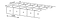Figure 1. Pixels of a row of an image with three channels. The numbers shown are the indices.

A pointer to an array of ints `xofs` points to an array that contains the starting indices of pixels on a row to be averaged in a later stage. For example, suppose we want to shrink the image to its third in its width and height. Then `iscale_x = 3`, and `iscale_y = 3`. What does `xofs` contains? They are 0, 1, 2, 9, 10, 11, etc. Notice the pattern? We take all the indices in the channel direction every `iscale_x` columns. It will be seen next that pixels with indices of 3, 4, 5, 6, 7, 8 will be combined with those of 0, 1, 2 to calculate a weighted average. So `xofs` marks the starting boundaries of all such averaging blocks.

We then calculate a variable `area`, which is equal to `iscale_x * iscale_y`. In our example, `area = 9`.

Another pointer to array of ints `ofs` points to an array of `area` number of indices. These indices are the offsets in x direction and y direction, such that they form a window for each channel, and the number of pixels in this window equals `area`. In our example, `ofs` points to an array of 9 elements. Suppose our original image has a width of 9 pixels. Then each row of the image has 27 pixels, taking account of 3 channels. Therefore, the first three indices of `ofs` are 0, 3, 6. And then the next three are 27, 30, 33. Then finally the last three are 54, 57, 60. This is illustrated in Figure 2 (Of course, the width of the image in the figure is not 9…).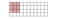Figure 2. A portion of an image. If iscale_x is 3 and iscale_y is 3, then the window is a 3-by-3 block.

With this window, we sum the values of the pixels within it, and then divided by `area`. The result is the pixel value of the output image. In other words, the algorithm simply calculates the average value of the boxed pixels. This averaging is done for each index in `xofs`, finishing each channel.

Quite intuitive and straightforward, isn’t it?

That’s for scales of integer value. For non-integer shrinking, the idea is still the same, but weight will change depending on how much a pixel in the original image is included in a window (note that this time the window size has decimal values). For a row of pixels, this can be seen from Figure 3, where different colors represent which pixels are included each time the window moves. The same thing applies to y direction.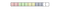Figure 3. The scale is not an integer, therefore the window size is not an integer. Different colors represent which pixels are included each time the window moves.

The weight of each pixel is the proportion that is included times `1/area`. For example, if 0.3 of pixel is contained in the window, then its weight is 0.3/area.

We can check it with a simple example:

`import numpy as npimport cv2# Create a numpy array, pretending it is our imageimg = np.array([[3, 106, 107, 40, 148, 112, 254, 151],                [62, 173, 91, 93, 33, 111, 139, 25],                [99, 137, 80, 231, 101, 204, 74, 219],                [240, 173, 85, 14, 40, 230, 160, 152],                [230, 200, 177, 149, 173, 239, 103, 74],                [19, 50, 209, 82, 241, 103, 3, 87],                [252, 191, 55, 154, 171, 107, 6, 123],                [7, 101, 168, 85, 115, 103, 32, 11]],                dtype=np.uint8)# Resize the width and height in halfresized = cv2.resize(img, (img.shape/2, img.shape/2),                     interpolation=cv2.INTER_AREA)print(resized)# Result:# [[ 86  83 101 142]# [162 103 144 151]# [125 154 189  67]# [138 116 124  43]]`

# Enlarging images

When we are enlarging an image with `INTER_AREA`, it is actually calling the bilinear function, but with a different way of calculating the interpolation coefficents.

For the bilinear method, assuming we consider a 1D image for simplicity, the interpolated pixel value is a weighted average of the two original neighbourhood pixels. The weights depend on the distances to the two pixels. Closeness means higher weight. And the weight values vary linearly with the distances. But before we continue, I’d like to talk about how the coefficients (weights) are actually calculated in OpenCV.

For a beginner like me, I would’ve thought that scaling a row like `[0, 1]` to 4 columns would be `[0, 0.3, 0.6, 1]`. However, this is not the behaviour of OpenCV’s `resize`. Nor is MATLAB’s `imresize`.

The code for calculating the coefficients in the x direction for the bilinear method in OpenCV is, for each pixel index `dx` of the output image,

`fx = (float)((dx+0.5)*scale_x - 0.5);    // (1)sx = cvFloor(fx);                        // (2)fx -= sx;                                // (3)`

Recall that `scale_x` is the ratio between the input image width and the output image width. In (1), the `fx` is the “floating-point” pixel index of the input image in the x direction, whereas in (3), the `fx` is the interpolation coefficient for the right pixel in the x direction (i.e., the interpolation coefficients are the pair `1-fx` and `fx`). So where does the equation in (1) come from?

One can image a 1D image in the following way shown in Figure 4.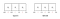Figure 4. Coordinates (indices) system of a 1D image. Boundaries and pixels all have separate coodinates. We assume pixels locate in the middle of boxes.

In Figure 4, we show the coordinate systems for a 1D image. We assume the pixels locate in the middle of pixel boxes, and boundaries have their own coordinates. Note that in MATLAB, index starts from 1.

Now what (1) does is to map the coordinates from the output image to the original input image. Since this is linear interpolation, to get the linear function, we need two equations. The first equation comes from the requirement that the left boundaries of the input and output images have the same coordinates. So that means -0.5 in the output image coordinate system should be mapped to -0.5 in the input image coordinate system. The second is that a distance of `inv_scale_x` in the output image coordinate system should be 1 in the input image coordinate system. Solving for the linear function, we then have the expression of (1). The MATLAB code for this mapping is written as

`u = x/scale + 0.5 * (1 - 1/scale);`

which can be found in `images.internal.resize.contributions`. Note that in the MATLAB code, `x` is the output image coordinate, `u` the input image coordinate and `scale` is the ratio of output image width over input image width, the same as `inv_scale_x` in OpenCV. So `[0, 1]` would actually become `[0, 0.25, 0.75, 1]`. Figure 5 shows the coefficients for the left pixel for 100 `dx`s when the output image width is two times of the input image.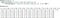Figure 5. The interpolation coefficients for the left pixel, calculated for 100 dx positions when the output image width is two times of the input image.

However, `INTER_AREA` has a different strategy for calculating the weights. And the behaviour is also slightly different depending on whether the scale factor is an integer or not.

The code for calculating the interpolation coefficient (the weight) in x direction is, for each pixel position of the output image `dx`:

`sx = cvFloor(dx*scale_x);fx = (float)((dx+1) - (sx+1)*inv_scale_x);            fx = fx <= 0 ? 0.f : fx - cvFloor(fx);`

and

`cbuf = 1.f - fx;            cbuf = fx;`

Calculation in y direction is the same. Here, `cbuf` contains the actual coefficients, where the first applies to the left pixel, and the second to the right pixel.

Since we are assuming `inv_scale_x` is an integer, then `fx` is essentially equivalent to

`int inv_scale_x_integer = saturate_cast<int>(inv_scale_x);fx = dx % inv_scale_x_integer + 1 - inv_scale_x_integer;`

We can see that `fx` cannot be larger than 1. In fact, the largest possible value is 0. Plus this statement:

`fx = fx <= 0 ? 0.f : fx - cvFloor(fx);`

This means, when `inv_scale_x` is an integer, `fx` is always 0, and the coefficients are always 1 and 0. This also means that the interpolated value is a copy of the left pixel. We can see this with a simple test:

`img = np.array([[ 86  83 101 142]                [162 103 144 151]                [125 154 189  67]                [138 116 124  43]], dtype=np.uint8)enlarged = cv2.resize(img, (8, 8), interpolation=cv2.INTER_AREA)print(enlarged)# Result:#[[ 86  86  83  83 101 101 142 142]# [ 86  86  83  83 101 101 142 142]# [162 162 103 103 144 144 151 151]# [162 162 103 103 144 144 151 151]# [125 125 154 154 189 189  67  67]# [125 125 154 154 189 189  67  67]# [138 138 116 116 124 124  43  43]# [138 138 116 116 124 124  43  43]]`

If `inv_scale_x` or `inv_scale_y` is not an integer, then the interpolation coefficients are no longer just 1 and 0. It is still similar to the integer case. It is just that the modulo is done with a real number. Although it is not well defined, we can still make a sense. Figure 6 shows the coefficients for the left pixel, calculated at 100 `dx` positions, with a `inv_scale_x` being 5.6: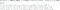Figure 6. The interpolation coefficients for the left pixel calculated at 100 dx positions, with a scale factor of 5.6.

Observe the pattern. Our scale factor is 5.6. Image that we are assigning values to each position, and at each position, the maximum amount we can assign is 1. Then from the beginning, we take 5 ones, and then there are only 5.6–5=0.6 to take, so the next coefficient is 0.6. After that, we have another 5.6 to divide. Last time we have 0.4 left to be filled up. We take that amount from the new 5.6, as if making the previous 0.6 to be 1. Now we keep assigning values so that at each position we have assigned the full amount, which is 1. Then after assigning another 5 ones, we have only 0.2 left. So the next one is 0.2.

# Summary

To sum up,

• When the output image is not larger than the input image both in width and height:

— The input/output scales in both width and height are integers:

1. If width and height are shrinked by half, and the number of channels is not 2, then `INTER_LINEAR_EXACT` is `INTER_AREA`;
2. If width and height are shrinked by half, then `INTER_LINEAR` is `INTER_AREA`;

`INTER_AREA` is the boxed/window resampling.

• When the output image is larger than the input image in either width or/and height:

— The output/input scales in both width and height are integers:

`INTER_AREA` is a bilinear interpolation with coefficients (1, 0).

— Otherwise:

`INTER_AREA` is a bilinear interpolation with slightly more complicated coefficient values.

Welcome to a place where words matter. On Medium, smart voices and original ideas take center stage - with no ads in sight. Watch
Follow all the topics you care about, and we’ll deliver the best stories for you to your homepage and inbox. Explore
Get unlimited access to the best stories on Medium — and support writers while you’re at it. Just \$5/month. Upgrade